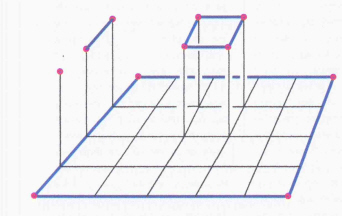Perturbation trick in the proof of Seifert-van-Kampen

The theorem of Seifert-Van-Kampen states that the fundamental group $\pi_1$ commutes with certain colimits. There is a beautiful and conceptual proof in Peter May's "A Concise Course in Algebraic Topology", stating the Theorem first for groupoids and then gives a formal argument how to deduce the result for $\pi_1$. However, there we need the assumption that our space is covered by path-connected open subsets, whose finite intersections are path-connected again. This assumption can be weakened using a little "perturbation" trick which is explained in the proof given by Hatcher in his book "Algebraic Topology". After doing this, we only need that triple intersections are path connected (and we cannot do better).

Question 1. Is it possible to "conceptualize" the perturbation trick, thus weakening the assumption concerning finite intersections? Maybe this is answered by one of the pure categorical proofs of Seifert-van-Kampen?

Question 2. In practice (explicit calculations of fundamental groups), do we actually often need or use that this weakening of the assumption is possible?

• since you haven't received any responses at MATH.SE, I suggest you to pose this question at MO
– user9413
Jul 3 '11 at 10:51

Question 1: One of the easiest ways to see this 3-fold intersection condition is in terms of "brick subdivisions" of the square. This is a subdivision of the square into rectangles such that each vertex of the subdivision is a corner of 3-rectangles. Such a subdivision can be taken fine enough to carry out the argument given in Hatcher's book. More details are in

R. Brown and A. Razak Salleh, A van Kampen theorem for unions of non-connected spaces'', Archiv. Math. 42 (1984) 85-88.

which relates this argument to the Lebesgue covering dimension. Also by using the fundamental groupoid $\pi_1(X,A)$ on a set $A$ of base points, it gives a theorem for the union of non-connected spaces.

I also prefer the argument in terms of "verifying the universal property" rather than looking at relations defining the kernel of a morphism. To see this proof in the case of the union of 2 sets, see

https://groupoids.org.uk/pdffiles/vKT-proof.pdf

One of the problems in the proof for the fundamental group as given by May is that it does not generalise to higher dimensions.

Question 2: One has to ask: where do such unions of non-connected spaces arise? The standard answer, and for me from which all this groupoid work arose, is to obtain the fundamental group of the circle $S^1$, which is after all, THE basic example in algebraic topology. Many other examples arise in geometric topology.

More answers come in applications to group theory; for example the Kurosh subgroup theorem on subgroups of free products of groups can be proved by using a covering space of a one-point union of spaces. The cover over each space of this union has usually many components. I confess I have not seen the minimal condition of 3-fold intersection used in practice, but it is always interesting to know the minimal conditions for a theorem. Just as well to know one can't in general get away with 2-fold intersections.

You can see the consistent use of groupoids in $1$-dimensional homotopy theory (van Kampen theorem, homotopy theory, covering spaces, orbit spaces) in my book Topology and Groupoids (2006) available from amazon.

Edit Feb 17, 2014:

It may be useful to point out that a sharper result than that in May's book was published in 1984 in the above Brown-Razak Salleh paper; the idea of proving a pushout result for the full fundamental groupoid, and then retracting to $\pi_1(X,A)$, is in my 1967 paper, and subsequent book, but Peter May cleverly makes it work for infinite covers; the problem is that this proof does not generalise to higher dimensions, as far as I can see.

Also Munkres' book on "Topology" uses non path connected spaces in dealing with the Jordan Curve Theorem, and for this uses covering space rather than groupoid arguments. I do not see why books avoid $\pi_1(X,A)$, since it hardly requires any extra in the proofs to that for $\pi_1(X,a)$, given the easy definition.

Edit Feb 20, 2015: A relevant question and answer is https://mathoverflow.net/questions/40945/compelling-evidence-that-two-basepoints-are-better-than-one

Some of the relevance to the history of algebraic topology, and to further developments, is in this presentation in Galway, December, 2014. See also my preprint page.

March 5, 2015I feel this "perturbation (or deformation) trick" is of quite a fundamental nature. Above is a picture of part of a deformation involved in the proof of the 2-dimensional Seifert-van Kampen type theorem, for the crossed module involving a triple $(X,A,C)$ of spaces where $C$ is a set of base points, and $C \subseteq A \subseteq X$.

The red dots denote points of $C$. The blue lines denote paths lying in $A$. The small squares in the bottom are supposed to denote squares lying in a set $U$ of an open cover. By making connectivity assumptions you can deform a small bottom square into the top one of the right kind, and still lying in the same open set. So, by working with a double groupoid construction $\rho_2(X,A,C)$ whose compositions are more 2-directional than the usual relative homotopy groups, you can get going with the proof of a universal property involving double groupoids, and hence for crossed modules.